Next: Effect of the Magnetic Up: ``Ecology'' of Magnetic Rotators Previous: The Stopping Radius

## The Stopping Radius in the Supercritical Case

Ā

The estimates presented above for the stopping radius were obtained under the assumption that the energy released during accretion does not exceed the Eddington limit,Ā so we neglected the reverse action of radiation on the accretion flux parameters.

Now, we turn to the situation where one cannot neglect the radiation pressure. Consider this effect after Lipunov (1982b). Suppose that the accretionĀ rate of matter captured by the magnetic rotator is such that the luminosity at the stopping radiusĀ exceeds the Eddington limit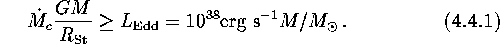We shall assume (after Shakura and Sunyaev, 1973) that the radiation sweeps away exactly that amount of matter which is needed for the accretion luminosityĀ of the remaining flux to be of the order of the Eddington luminosityĀ at any radius: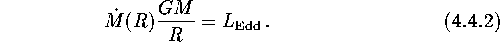This gives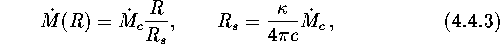where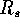is a spherization radiusĀ (where the accretion luminosity first reaches the Eddington limit),Ā anddesignates the specific opacity of matter. Using the continuity equation, the ram pressure of the accreting plasma is now obtained as another function of the radial distance (see FigureĀ4)in contrast to the subcritical regime when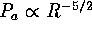.

Matchingand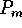(see the previous section) for the supercritical case gives (see inequality (4.4.6))

Ā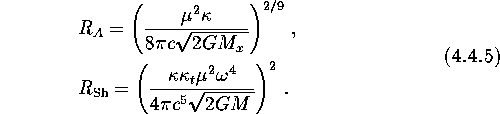The critical accretion rate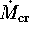is defined by the boundary of the inequality

Ā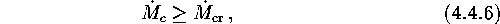and, correspondingly, is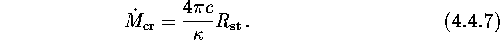The dependence of the Alfven radiusĀ on the accretion rate is such that the Alfven radius (beyond the capture radius) slightly decreases with increasing accretion rate as, while it decerases below the capture radius asand attains its lowest value (4.4.5) for the critical accretion rate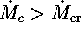, beyond which it is independent of the external conditions.

We also note that in the supercritical regime, the pressure of the accreting plasma increases more slowly (as) when approaching the magnetic rotator than the pressure of the relativistic wind (as) ejected by it. This means that in the supercritical case a cavernĀ may exist even below the capture radius.Ā

The estimates presented here, of course, are most suitable for the case of disk accretion. Ā In fact, the supercritical regime seems to emerge most frequently under these conditions. This can be simply understood. Indeed, the accretion rate is proportional to the square of the capture radius. At the same time, the angular momentum of the captured matter is also proportional to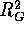. Hence, at high accretion rates the formation of the disk looks natural.ĀNext: Effect of the Magnetic Up: ``Ecology'' of Magnetic Rotators Previous: The Stopping Radius

Mike E. Prokhorov
Sat Feb 22 18:38:13 MSK 1997